• 模式识别人工神经网络BP算法
千次阅读
2020-09-15 18:30:38

识别活动是人类的基本活动，人们希望机器能代替人类进行识别工作。因此模式识别的理论和方法引起了人们极大的兴趣并进行了长期的研究，现已发展成一门多学科交叉的学科。针对不同的对象和不同的目的，可以用不同的模式识别理论、方法。而人工神经网络能较好地模拟人的形象思维，而且由于具有大规模并行协同处理能力、较强的容错能力和联想能力以及较强的学习能力，所以将神经网络方法运用到模式识别中去解决识别问题己成为国内外科技工作者广泛关注的热点。

本文首先对模式识别、神经网络的发展概况以及应用神经网络来进行模式识别的历史渊源等方面作了较为详细的介绍，然后分析了模式识别的一些基本概念、方法，指出了其困难和要求，同时给出了神经网络进行模式识别的方法，并比较它与传统方法的异同，说明了神经网络方法进行模式识别的特点与优越性；然后给出了本文核心即BP神经网络模式分类的实现思想。运用上述研究成果，借助于MATLAB，用BP算法完成了神经网络结构设计。进行的测试表明其运行稳定、字符识别率较高，表明了本文研发成果的有效性。

神经网络的研究是以人脑为基础的一门智能科学的研究与探索，实际上，40年代初，就有人认识到这一领域研究的重要性。可以说，它的研究与当今串行计算机的研究是同步进行的。但是，由于当时分子生物学发展的限制，人脑的机理还未真正弄清楚，从而影响这一研究领域的进展:另一方面，由于VLSI技术日新月异的进步，使串行计算机及人工智能技术在规模、速度上得到迅猛的发展，从而掩盖了这一科学研究的必要性和迫切性。所以，当时神经网络理论的发展是缓慢的。

80年代，人们逐渐认识到，由于传统计算机是模式识别
更多相关内容
• 论文研究-人工神经网络BP算法的数据处理方法及应用.pdf, 利用人工神经网络建立了具有时间序列的对象的预测模型，并提出了基于本模型的数据处理方法，在此基础上，对吉林...
• 基于人工神经网络BP算法的黄冈市房价预测.pdf
• 基于人工神经网络BP算法的莆田市房价预测.pdf
• 人工神经网络BP算法在四川省GDP预测中的应用.doc
•文档
• 人工神经网络BP算法。使用神经网络时使用误差逆传播(简称BP)算法进行训练。误差逆传播算法是迄今为止最成功的神经网络学习算法。BP算法的工作流程如图所示：1、对每个训练样例，先将输入示例提供给输入层，然后逐层...

# 动图很长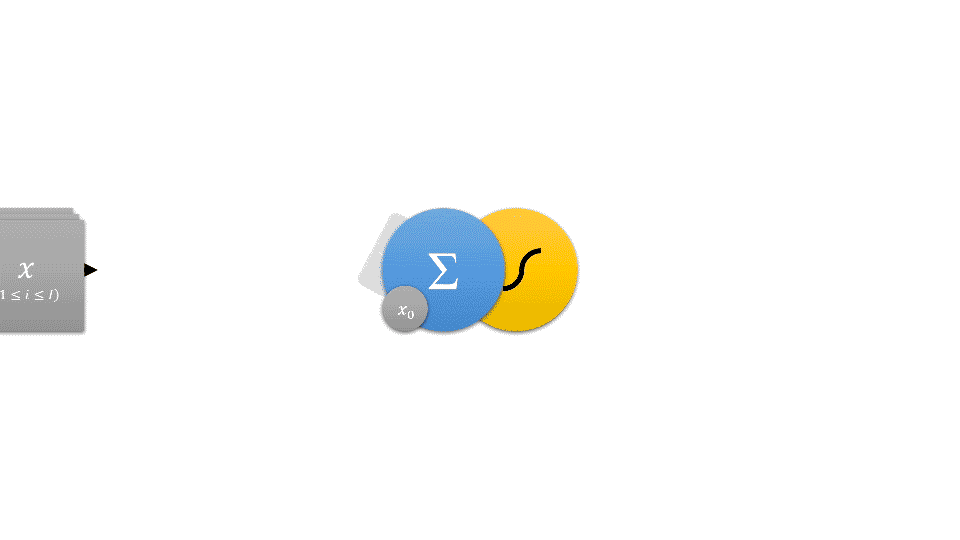# 代码

# -*- coding: utf-8 -*-

import numpy as np
import matplotlib.pyplot as pyp
OUTPUT = 'output'
SUM = 'sum'
INPUT = 'input'
EXPECT = 'exp'
WEIGHT = 'weight'
LOST = 'lost'
START = 'start'
END = 'end'
B = 1.0  # 额外的输入

def af(v):
"""激励函数。

Args:
v (matrix): 自变量

Returns:
matrix: 函数值。
"""
return 1/(1+np.exp(-v))

def af_d(v):
"""激励函数的导数。

Args:
v (matrix): 自变量

Returns:
matrix: 函数值。
"""
d = af(v)
return np.multiply(d, 1.0-d)

def loss(y_hat, y):  # 交叉熵损失函数L(y^,y)=-ylog(y^)-(1-y)log(1-y^)
"""交叉熵损失函数。

Args:
y_hat (matrix): 观测结果即神经网络输出层返回值
y (matrix): 模型输出

Returns:
float: 计算模型输出和观测结果间的差异。
"""
ret = []
row_count, col_count = y_hat.shape
for row_index in range(row_count):
y_hat_row_t = y_hat[row_index, ].T
left = np.dot(-y, np.log(y_hat_row_t))
right = np.dot((np.array([]) - y), np.log(1 - y_hat_row_t))
res = np.sum(left-right)
ret.append(res)
pass
return ret  # -np.sum(y_hat*np.log(y))

def init_weights(*widths):
"""初始化权值。

Returns:
list: 初始权值。
"""
weights = []
depth = len(widths)
for i in range(1, depth):
miu = np.sqrt(1/widths[i])  # 权值方差，控制权重值分布不要太零散
w = miu * np.random.randn(widths[i], widths[i-1]+1)  # 随机初始化
weights.append(np.mat(w))
pass
return weights  # 长度为神经网络深度-1(减去输入层)，每层行数为本层宽度n，列数为前层宽度m+1(加上偏置值)

def fp_layer(input, weights):
"""单层前向传播。

Args:
input (matrix): 本层输入值，每一行表示对前一层一个神经元的输出
weights (matrix): 本层权值

Returns:
dict: 包含本层输入值(包含额外输入)，本层加权求和和非线性变换结果。
"""
iab = np.insert(np.mat([[B]]), , input, axis=0)  # 加入偏置于input头部
sums = np.dot(weights, iab) # 加权求和
res = af(sums) # 非线性变换
return {INPUT: iab, SUM: sums, OUTPUT: res}

def bp_layer(exp, weights, sum, inputs):
"""单层BP

Args:
exp (matrix)): 本层模型输出，每一行表示本层一个神经元的模型输出
weights (matrix): 本层权值
sum (matrix): 前层加权求和结果
inputs (matrix): 本层输入值(包含额外输入)

Returns:
dict: 前层模型输出和权值变化量。
"""
# exp为n*1
delta_weights = np.dot(exp, inputs.T)  # 没乘学习率
propagate = np.dot(weights.T[1:], exp) # 偏置列不传播
propagate = np.multiply(grad, propagate) # δ=f'(s)(w^T*δ^+1)
return{EXPECT: propagate, WEIGHT: delta_weights}

def fit(data, weights):
"""对单个数据拟合。

Args:
data (dict): 包含输入和模型输出
weights (list): 神经网络权值

Returns:
dict: 包含观测结果和权值变化量。
"""
depth = len(weights)
fp_results = []
input = data[INPUT]
for i in range(depth):
fp_res = fp_layer(input, weights[i])  # 前向传播
fp_results.append(fp_res)
input = fp_res[OUTPUT]
pass
delta_weights = []
exp = input - data[EXPECT] # y-y_hat
for i in reversed(range(depth)):
net = fp_results[i-1][SUM]
bp_res = bp_layer(exp, weights[i], net, fp_results[i][INPUT])  # BP
exp = bp_res[EXPECT] # 反向传播
delta_weights.append(bp_res[WEIGHT]) # 保存ΔW
pass
delta_weights.reverse()  # 权重层序号是反的，需要反转，此处反转
return{OUTPUT: input, WEIGHT: delta_weights}

times = 3000
eta = 0.25  # 学习率
data_inputs = [[1, 0], [1, 1], [0, 1], [0, 0]] # 一个异或的数据
data_outputs = [, , , ]

data_size = len(data_inputs)
weights = init_weights(2, 3, 1)
depth = len(weights)
result = {LOST: [], START: None, END: []}
for t in range(times):
delta_weights = []
res = []
for data_index in range(data_size):  # 拟合每一组数据
data = {INPUT: np.mat([data_inputs[data_index]]).T,
EXPECT: np.mat([data_outputs[data_index]]).T}
fit_res = fit(data, weights)

delta_weights.append(fit_res[WEIGHT])
res.append(fit_res[OUTPUT].T.tolist())  # 之后res就不参加运算了
pass

if result[START] is None:  # 存储神经网络输出以供打印
result[START] = res
result[END] = res

lost = loss(np.mat(res).T, np.mat(data_outputs).T)
result[LOST].append(np.dot(1/data_size, lost))

for delta_weight in delta_weights:
for layerIndex in range(depth):
layer = delta_weight[layerIndex]
weights[layerIndex] -= np.dot(eta, layer)  # 更新权值和乘学习率
pass
pass
pass

print('\033[31m', '训练前输出：', np.mat(result[START]).T, '\033[0m')
print('\033[35m', '训练后输出：', np.mat(result[END]).T, '\033[0m')
pyp.plot(result[LOST]) # 画图看损失
pyp.show() # 展示图


# 输出

 训练前输出： [[0.57172222 0.55289663 0.61069527 0.63006013]]
训练后输出： [[0.99686652 0.00336393 0.9959174  0.00293138]]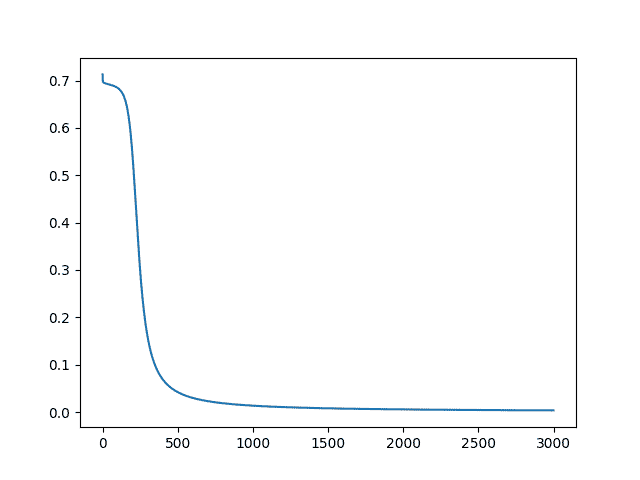# 用tensorflow实现代码更短：戳我跳转

展开全文python 机器学习
• 使用java语言描述bp神经网络算法，该算法比较容易让人理解。
• 人工神经网络BP算法在四川省 GDP预测中的应用 作者姓名XXX 专业班级数学与应用数学 指导教师XXX 摘 要 GDP与大家生活息息相关没有GDP人们便无法反映一国的贫富状况和人民的平均生活水平无法确定一国承担的国际义务...
• 通用的人工神经网络BP算法程序，包含程序设计步骤及源代码。
• 人工神经网络BP算法的理解及如何利用神经网络挑瓜人工神经网络的理解：BP算法的理解：5.13公式的推导：模板代码： 人工神经网络的理解： 在开始看到人工神经网络时，自以为是很厉害的东西，但随着了解的深入，发现...

# 一. 人工神经网络的理解：

在开始看到人工神经网络时，自以为是很厉害的东西，但随着了解的深入，发现其实也就是那点东西，说白了就是套模板的事。这里要和生物神经网络做区分，虽然人工神经网络也叫神经网络，但其复杂度远不如生物的神经网络，这里是通过代码进行模拟，即并没有创建所谓的神经元，而是用一些参数来假设神经元的存在，例如创建两个神经元之间的的路径的数值及其他特征的数值来模拟神经元的存在.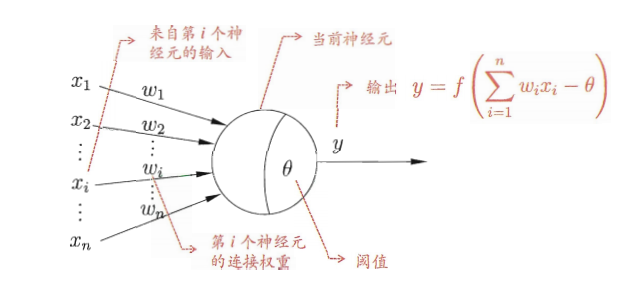这里将一个神经元看作一个函数，它具有输入和输出。而输入值具有多个，我们对每一个输入值进行一个赋权操作，然后令赋权后值相加，把相加的和与该神经元的阈值做差，将差值作为该神经元的最终的输入，简单来说就是对初始的每一个变量乘一个系数，然后相加。之后把最终的输入值当作自变量，算出该神经元所对应的函数的值，该神经元所对应的函数是人为设定的，一般采用这个函数sidmoid（图像如下）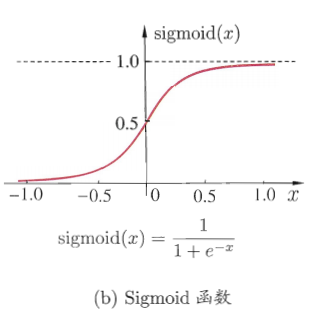采用这个函数是因为这个函数是连续的，而且具有很好的求导性质，即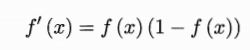对于之后进行权值和阈值更新比较方便

在建立神经网络时，首先建立输入层和输出层，然后根据需要，建立隐层。隐层的数量可以为多层，往往在建立时采用一层隐层，这是因为一层的准确度已经比较高了，采用多层会增加运算上的负担，同时准确率提升不高，当然，也可以不建立隐层，只用输入层和输出层，对于一些简单的处理已经足够了。

以下是两个神经网络图模型：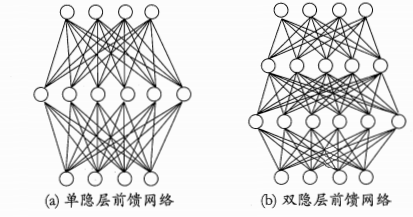# 二. BP算法的理解：

BP算法即误差逆传播算法，先设定神经网络的初始权值和阈值，然后把输入值输入神经网络的输入层，然后令其通过神经网络的处理后得到最终的结果，将这个结果与结果标签进行对比，然后更新这个神经网络的阈值和权值，使得实际的结果去向结果标签靠近。每经过一个样例便进行一次调整，可以认为，当调整的次数足够多时，该神环境网络的阈值和权值已经变得非常理想，此时，该神环境网络的错误率变得非常低，即我们所谓已经训练好的人工智能，我们可以将这个训练好的神环境网络去处理其他数据，进而得出预期的结果。

这里以单个隐层的神经网络为例，假设输出层有L个神经元，我们采用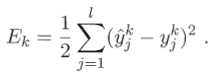Ek叫做均方误差。
理想话的情况Ek的值为0，然而实际值不为零，则实际值与与预期值的差即为Ek，通过该值去调整权值和阈值。

# 三. 5.13公式的推导：

这里进行资料5.13式的推导，先给出已经推导好的公式

输出层第J个神经元的阈值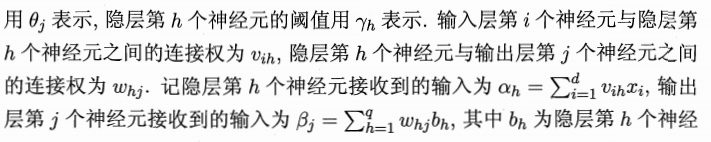元的输出。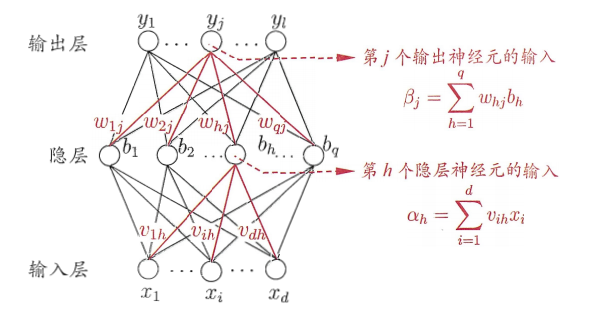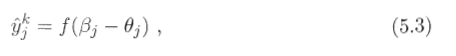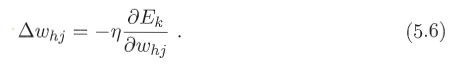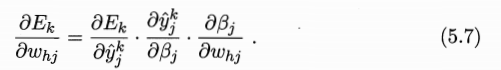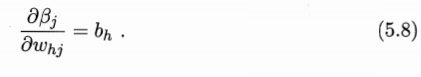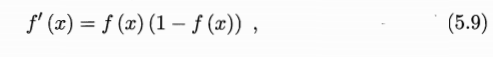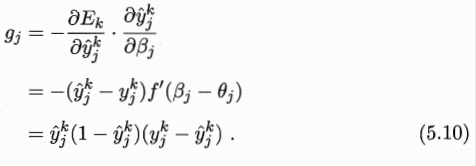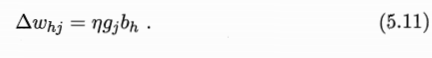基于上述推导，我们推导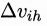根据定义可知：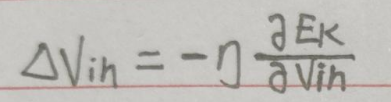其中：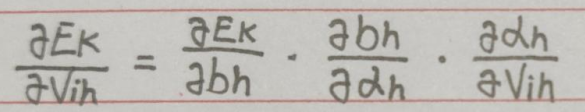我们对这三个分别求:
首先：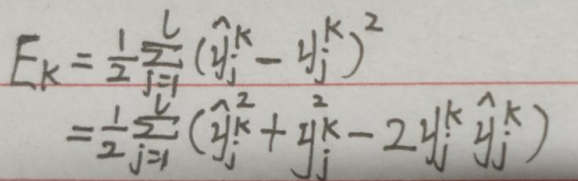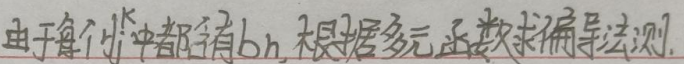则：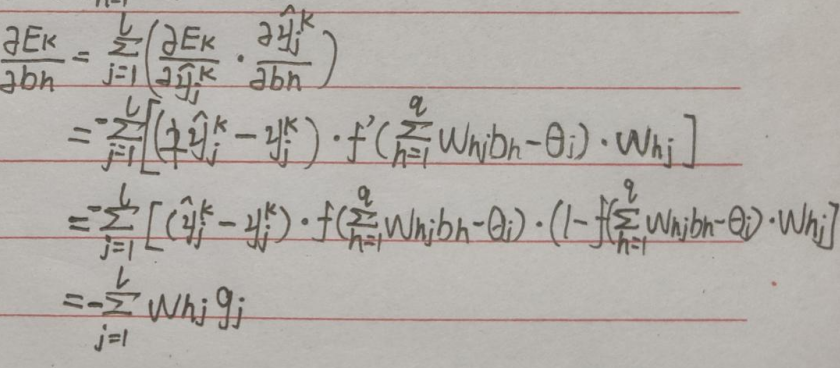gi为之前已经推导出的
之后求第二个：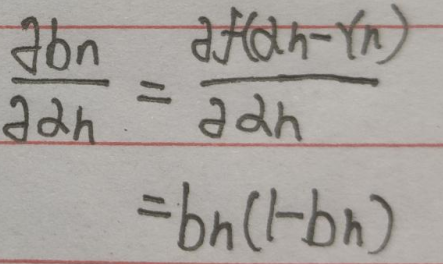这里用到了：再求最后一项：
这个比较简单：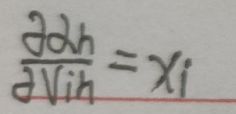综上：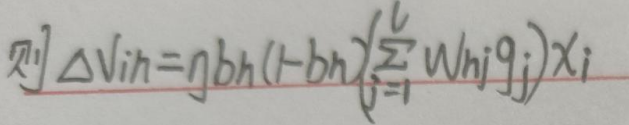# 四. 模板代码：

import numpy as np
from numpy.core.fromnumeric import put
from numpy.lib.function_base import append
import pandas as pd
from pandas.core.construction import array
from sklearn import preprocessing

# 设置文本标签转数字程序
trans1 = preprocessing.LabelEncoder()
trans2 = preprocessing.LabelEncoder()
trans1 = trans1.fit([
"青绿", "乌黑", "浅白", "蜷缩", "稍蜷", "硬挺",
"浊响", "沉闷", "清脆",
"清晰", "稍糊", "模糊", "浊响",
"平坦", "凹陷", "稍凹",
"硬滑", "软粘"
])
trans2 = trans2.fit(["是", "否"])
# 加载数据

# path = "C:\\Users\\小白白\\Desktop\\names.txt"
file = open(path, "r", encoding="utf8")
# 存放数据
dataset = np.array([])
# 存放标签
labelset = np.array([])
a = i.strip().split(",")
temp = []
temp.extend(a[1:-3])
temp = trans1.transform(temp)
temp = np.append(temp, a[-2])
temp = np.append(temp, a[-3])
dataset = np.append(dataset, temp)
p = np.array([])
p = np.append(p, a[-1])
p = trans2.transform(p)
labelset = np.append(labelset, p)
# 每个数据行的最后一个是标签
dataset = dataset.reshape((17, 8))
file.close()
return dataset, labelset

def parameter_initialization(x, y, z):

# 隐层阈值
value1 = np.random.randint(-5, 5, (1, y)).astype(np.float64)

# 输出层阈值
value2 = np.random.randint(-5, 5, (1, z)).astype(np.float64)

# 输入层与隐层的连接权重
weight1 = np.random.randint(-5, 5, (x, y)).astype(np.float64)

# 隐层与输出层的连接权重
weight2 = np.random.randint(-5, 5, (y, z)).astype(np.float64)
'''value1=np.ones((1,8))
value2=np.ones((1,1))
weight1=np.ones((8,8))
weight2=np.ones((8,1))'''
return weight1, weight2, value1, value2

def sigmoid(z):
return 1 / (1 + np.exp(-z))

def trainning(dataset, labelset, weight1, weight2, value1, value2):
# x为步长
x = 0.1
for i in range(len(dataset)):
# 输入数据
inputset = np.mat(dataset[i]).astype(np.float64)
# 数据标签
outputset = np.mat(labelset[i]).astype(np.float64)
# 隐层输入
input1 = np.dot(inputset, weight1).astype(np.float64)
# 隐层输出
output2 = sigmoid(input1 - value1).astype(np.float64)
# 输出层输入
input2 = np.dot(output2, weight2).astype(np.float64)
# 输出层输出
output3 = sigmoid(input2 - value2).astype(np.float64)

# 更新公式由矩阵运算表示
a = np.multiply(output3, 1 - output3)
g = np.multiply(a, outputset - output3)
b = np.dot(g, np.transpose(weight2))
c = np.multiply(output2, 1 - output2)
e = np.multiply(b, c)

value1_change = -x * e
value2_change = -x * g
weight1_change = x * np.dot(np.transpose(inputset), e)
weight2_change = x * np.dot(np.transpose(output2), g)

# 更新参数
value1 += value1_change
value2 += value2_change
weight1 += weight1_change
weight2 += weight2_change
return weight1, weight2, value1, value2

def testing(dataset, labelset, weight1, weight2, value1, value2):
# 记录预测正确的个数
rightcount = 0
for i in range(len(dataset)):
# 计算每一个样例通过该神经网路后的预测值
inputset = np.mat(dataset[i]).astype(np.float64)
outputset = np.mat(labelset[i]).astype(np.float64)
output2 = sigmoid(np.dot(inputset, weight1) - value1)
output3 = sigmoid(np.dot(output2, weight2) - value2)

# 确定其预测标签
if output3 > 0.5:
flag = 1
else:
flag = 0
if labelset[i] == flag:
rightcount += 1
# 输出预测结果
print("预测为%d   实际为%d" % (flag, labelset[i]))
# 返回正确率
return rightcount / len(dataset)

filename = "C:\\Users\\小白白\\Desktop\\names.txt"
w1, w2, v1, v2 = parameter_initialization(len(d1), len(d1), 1)
for i in range(5000):
w1, w2, v1, v2 = trainning(d1, d2, w1, w2, v1, v2)
sum=0
for i in range(10):
sum+=(testing(d1,d2,w1,w2,v1,v2))
print(sum/10.0)
t = int(input("请输入测试样例个数："))
for i in range(t):
s = input("请按顺序输入西瓜特征:")
s = s.strip().split(",")
temp = []
temp.extend(s[1:-2])
temp = trans1.transform(temp)
temp = np.append(temp, s[-2])
temp = np.append(temp, s[-1])
inputset = np.mat(temp).astype(np.float64)
output2 = sigmoid(np.dot(inputset, w1) - v1)
output3 = sigmoid(np.dot(output2, w2) - v2)
p=""
if output3 > 0.5:
p = "好"
else:
p = "坏"
print("该西瓜判断为%s瓜" % p)



当然，学习率的设置要适当，通常设置为0.1；
学习次数越多，所得的准确率越高

由于初始设定的权值和阈值为随机产生的，所以准确率有一定的浮动，
每次运行程序所得的准确率都不同，但大致在一定范围内，所谓没必要太过计较所造成的偏差。

以下为训练集：

编号,色泽,根蒂,敲声,纹理,脐部,触感,密度,含糖率,好瓜
1,青绿,蜷缩,浊响,清晰,凹陷,硬滑,0.697,0.46,是
2,乌黑,蜷缩,沉闷,清晰,凹陷,硬滑,0.774,0.376,是
3,乌黑,蜷缩,浊响,清晰,凹陷,硬滑,0.634,0.264,是
4,青绿,蜷缩,沉闷,清晰,凹陷,硬滑,0.608,0.318,是
5,浅白,蜷缩,浊响,清晰,凹陷,硬滑,0.556,0.215,是
6,青绿,稍蜷,浊响,清晰,稍凹,软粘,0.403,0.237,是
7,乌黑,稍蜷,浊响,稍糊,稍凹,软粘,0.481,0.149,是
8,乌黑,稍蜷,浊响,清晰,稍凹,硬滑,0.437,0.211,是
9,乌黑,稍蜷,沉闷,稍糊,稍凹,硬滑,0.666,0.091,否
10,青绿,硬挺,清脆,清晰,平坦,软粘,0.243,0.267,否
11,浅白,硬挺,清脆,模糊,平坦,硬滑,0.245,0.057,否
12,浅白,蜷缩,浊响,模糊,平坦,软粘,0.343,0.099,否
13,青绿,稍蜷,浊响,稍糊,凹陷,硬滑,0.639,0.161,否
14,浅白,稍蜷,沉闷,稍糊,凹陷,硬滑,0.657,0.198,否
15,乌黑,稍蜷,浊响,清晰,稍凹,软粘,0.36,0.37,否
16,浅白,蜷缩,浊响,模糊,平坦,硬滑,0.593,0.042,否
17,青绿,蜷缩,沉闷,稍糊,稍凹,硬滑,0.719,0.103,否

展开全文python
• 非常好的人工神经网络bp算法异或 直接可以运行的mfc程序，很强大
• BP 算法 这是第一次尝试在csdn上写blog，主要因为我也是刚刚接触人工智能，所以各方面都在尝试中，所以想通过这种方式以讲代学，内...sigmoid 函数是人工智能神经网络中最常使用的一类激活函数，其数学表达式为： ...

# BP 算法

这是第一次尝试在csdn上写blog，主要是因为我也是刚刚接触人工智能，各方面都在摸索中，所以想通过这种方式以讲代学，这篇blog内有很多不足之处，希望各路大佬能够海涵

## 预备知识

在开始之前，首先需要补充一点预备知识。

### 1. 激活函数sigmoid函数：

sigmoid 函数是人工智能神经网络中最常使用的一类激活函数，其数学表达式为：sigmoid函数有一个重要的性质：f’(x) = f(x)[1-f(x)],这个性质在我们求导的过程中起了很大的作用。

### 2. 梯度下降策略其中，学习率一塔我们设定为0.5

接下来我们进入正题，BP算法总共分为两个部分，第一个部分是FP（前馈计算计算误差）过程，我们用来计算误差；第二个部分是BP（反馈计算更新权重），我们用来更新权重W；

## 一、 FP过程想必大家都已经了解到神经网络的结构，这里我就不做过多的解释了，其中l1, l2是这个神经网络的输入层，h1,h2,h3是这里的隐藏层，O1,O2是我这个神经网络的输出层，其中w表示权重，b表示偏置项（b可以理解为一次函数中的y = kx + b 中的常数项，在这里我们取b1 = 0.35, b2 = 0.65,其实 b1, b2 我们可以取任意值，在此不影响结论）。

为了方便更直观的解释，我们来设定一下权重值和偏置项的值：下面我们就来进行前馈计算：
首先我们以h1为例：

在此，我要补充一个知识：
一个神经元节点，它分为两个部分，如图所示：左边一部分是我们的输入值，我们称之为net(h1),net(h1)经过sigmoid函数激活之后，我们可以得到Out(h1)这就是我们隐藏层的一个输出结果。

下面我们来进行计算h1的误差：

net(h1) = w1 * l1 + w2 * l2 + b1=2.35

(你可以发现, h1是由w1和w2 连接而成的)
然后,经过sigmoid函数激活之后我们可以得到,

Out(h1) = sigmoid(net(h1))=0.912934

同理，可得：

Out(h2) = 0.979164
Out(h3) = 0.995275

有了这三个数值，我们可以算出输出层O1,O2的误差：

net(O1)= W7 * Outh1 + W9 * Outh1 + W11 * Outh3 + b2 = 2.1019206
经过sigmoid函数激活之后，我们可以得到：

Outh1 = sigmoid(neth1) = 0.891090

同理，可得：

Out h2 = 0.904330

然后，我们可以得到总误差，这里我们记作Etotal(这里我们将Out h1, Out h2 简称为 O1， O2):这里的1/2是为了后面反馈计算方便而添加的。
到此为止，我们已经完成了前馈计算，并且算出了总误差值。

## 二、 BP过程

### (1).更新（hidden layer ----- Output layer）

这里的BP过程，我们是用来更新W的值，使预测结果更加精确。在此，我们以W7为例。

这里我们需要用到高等数学里的链式法则，W7与下列因素相关：根据链式法则，我们可得：其中，等式右边第一项等于：第二项等于：第三项等于：所以，我们可以得到总式：最后，我们求得w7的更新结果：同理，我们可得：(w1-w6 求解方法见下)

## (2).更新（Input layer ----hidden layer）

这里我们以w1 为例：
这里还是应用高等数学里的链式法则，w1与下列因素有关：所以，我们得到求导公式：这里以Eo1对Outh1的求导为例：同理可得Eo2对h1的求导公式：所以我们求得更新后的w1的值：同理可得（w1 - w6）：至此，w的权重值已完成一次迭代周期。事实上，要想预估的更准确，我们需要上百次甚至上千次的迭代，直至我们权重最终的更新值图像对于实际值收敛。

# 代码实现：# 总结：

bp算法在我们现实中有很多应用，比如，根据某一商店的去年的销量，我们可以来预测今年的销量······我这里只做了简单的算法的数学推算，有许多不足之处，希望大家指正。

**最后：我要鸣谢b站

东方耀888

大佬，感谢大佬，有很多知识都是走他那边学习的！！

最后的最后： 感谢各位读者能耐心看完鄙人的文章！！万分感谢！！🙏**

展开全文机器学习
• 基于人工蜂群的BP神经网络 人工蜂群算法的反向传播神经网络。 基于人工蜂群算法的反向传播神经网络，通过大量尝试提出对神经网络误差调整参数进行优化的方法 BP神经网络 基于人工蜂群的BP神经网络
• 本文来自于csdn，本文是主要介绍了神经网络应用在分类问题中效果，以及神经网络结构及算法，希望对您的学习有所帮助。1.1基本结构说明：通常一个神经网络由一个inputlayer，多个hiddenlayer和一个outputlayer构成。...
• 一般的单级倒立摆神经网络控制，matlab仿真已实现，共同学习
• 人工神经网络是由大量的神经元按照大规模并行的方式通过一定的拓扑结构连接而成的。按照一定的规则将神经元连接成神经网络，并使网络中各神经元的连接权按一定的规则变化，这样一来也就产生了各式各样的神经网络模型...数据挖掘
• 10fx=0.12exp(-0.23x)+0.54*exp(-0.17x)*sin(1.23x)说明：1）网络结构为三层（输入层、1个隐层和输出层），隐层神经元个数自选； 2）获取两组数据，一组作为训练集，一组作为测试集； 3）用训练集训练网络； 4）用...
• 人工神经网络BP算法改进与分析，来源于中国知网，中国期刊，专业人士书写
• 人工神经网络BP算法在Microsoft Visual C+ + 610 下的实现
• 遗传算法BP人工神经网络算法C++实现代码是通过c++来 完成两个算法，里面有详细的注释说明，希望对你有用 遗传算法BP人工神经网络算法C++实现代码是通过c++来 完成两个算法，里面有详细的注释说明，希望对你有用
• 这是人工神经网络BP算法导论的课件，可以详细了解人工神经网络中的BP算法...